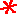Main      Site Guide### Scrambled Equations

• Scrambled Equations

In each of these puzzles, you are given a number that you must construct out of several other numbers. You do this by taking the numbers and performing addition, subtraction, multiplication, and/or division operations on them. Each number must be used in the calculations exactly once, and only these four operations listed may be used. You may parenthesize your expression however you wish. For example, 5 may be obtained from 1, 2, and 3, with the expression (3 + 2) × 1.

In many if not most cases, multiple solutions are possible, but usually only one is given on the solution page.

## #101Make 28 out of 4, 6, 8, and 8.

Hint

Solution

## #102

Make 55 out of 3, 3, 7, and 7.

Hint

Solution

## #103

Make 24 out of 3, 3, 7, and 7.

Hint

Solution

## #104Make 24 out of 1, 3, 4, and 6.

Hint

Solution

## #105

Make 44 out of 2, 4, 7, and 8.

Hint

Solution

## #106Make 383 out of 1, 2, 25, 50, 75, and 100.

Hint

Solution

## #107

Make 25 out of 3, 3, 6, and 9.

Hint

Solution

## #108Make 10 out of 3, 4, 7, and 8.

Hint

Solution

## #109

Make 7 out of 6, 8, 8, and 9.

Hint

Solution

## #110

Make 4 out of 2, 2, 2, and 9.

Hint

Solution

## #111

Make 1 out of 1, 7, 8, and 9.

Hint

Solution

## #112

Make 26 out of 3, 6, 7, and 8.

Hint

Solution

## #113

Make 14 out of 1, 5, 6, and 9.

Hint

Solution

## #114Make 39 out of 2, 5, 6, and 9.

Hint

Solution

## #115

Make 43 out of 1, 5, 6, and 9.

Hint

Solution

## #116

Make 33 out of 2, 5, 5, and 7.

Hint

Solution

## #117

Make 11 out of 4, 5, 7, and 7.

Hint

Solution

## #118

Make 31 out of 2, 5, 6, and 8.

Hint

Solution

## #119Make 22 out of 4, 5, 8, and 9.

Hint

Solution

## #120

Make 29 out of 2, 3, 6, and 8.

Hint

Solution

## #121

Make 33 out of 1, 2, 5, and 8.

Hint

Solution

## #122

Make 88 out of 1, 4, 7, and 9.

Hint

Solution

## #123

Make 25 out of 1, 4, 7, and 9.

Hint

Solution

## #124

Make 15 out of 1, 4, 7, and 9.

Hint

Solution

## #125

Make 7 out of 1, 1, 5, and 9.

Hint

Solution